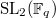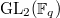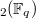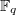﻿

### On the structure of Generalized Symmetric Spaces of$\bm{\mbox{SL}_{2}(\mathbb{F}_{\MakeLowercase{q}})}$} and$\bm{\mbox{GL}_{2}(\mathbb{F}_{\MakeLowercase{q}})}$

#### Abstract

In this paper we will discuss the generalized symmetric spaces for$\SL_2(\mathbb{F}_q)$ and$\GL_2(\mathbb{F}_q)$. Specifically we will characterize the structure of these spaces and prove that when the characteristic of$\mathbb{F}_q$ is not equal to two the extended generalized symmetric space is equal to the generalized symmetric space for$\SL_2(\mathbb{F}_q)$ and nearly equal for$\GL_2(\mathbb{F}_q)$ for all but one involution.

DOI Code: 10.1285/i15900932v37n2p1

Keywords: generalized symmetric space; special linear group; nite elds

Full Text: PDF